# The Retired Professor Simplifies Algebra: Exponents

## This is a free resource on understanding algebra, and presented in a short presentation aimed at making things clear. It may help thoses studying algebra and those trying to help.

Exponents must precede certain other topics, but has no predecessor, so we could have well started with exponents. However, in subsequent material both solving equations and proper use of exponents are both needed to work with such topics as polynomial equations beyond linear equations.

Proper use of exponents requires we adhere to the rules shown below. Understanding of these rues requires accepting definitions and gaining insight from using simple examples.

This segment of algebra can stand alone or can be used in material that will follow it.

### Exponents: Understanding the Rules

I have taught algebra many times, and one thing that is amazing is that exponents are presented several times.  There is a need for this.  Constraints happen as we expand out studies past positive integers, yet students often study as though the whole concept is new.

The rules for exponents are the same when they are presented numerus times in a textbook, except one cannot divide by 0, one is not to raise 0 to the 0-th power, and one cannot take as even root of a negative number in the real number system.  An even root occurs when we have a fractional power with an even number for the denominator, such as 51/2.  When one of the difficulties mentioned occurs, an author restates the same rules.  Learn them once and allow the authors to restate them.

In other words, when the rules for exponents are first encountered it may be for positive integers.  When this is extended to include the number zero, such as when all integers are considered, we must be careful to not divide by zero or have zero raised to the zeroth power.  Just follow the constraints of algebra and the rules learned once can still be used, with no fear something different is being added.

Let’s go through exponents once, learn the rules, and apply constraints where appropriate.  We will understand by example, which is not technically a proof.  Our examples will involve integers, but the same rules apply for fractions, and even complex numbers.

anam = an+m

8284 = 82 + 4 = 86

an/am = an m

75/72 = 75 – 2 = 73

a0 = 1, if a ≠ 0

an/an = 1, and the above rule makes this the same as a0.

a-n = 1/an

This is a definition.  It is equally correct to say an = 1/a-n

2-3 = 1/23

(an)m = anm

(52)3 = 52X3

(52)3 = (52)(52)(52) = (5X5)(5X5)(5X5) = 56

52X3 = 56

(ab)n=anbn

(4X3)2 = 4X3X4X3 = 4232

(a/b)n = anbn

(2/3)2 = (2/3)(2/3) = 22/32

Finally, we get the last two, which can be gotten from the above.

(ab)n(ab)man + mbn + m and (a/b)n(a/b)man + m/bn + m

### Simplification

An exponential expression is said to be simplified if there is a single number as the coefficient, each variable occurs no more than once, and only positive exponents are present.

Notice that there are many paths to the simplified form, one does not have to choose the order, or even the rules, shown.  There is much latitude in simplifying exponential expressions.

Simplification can be shortened by applying multiple rules in the same step, but I did it step by step so it would make sense to one not yet skipped in algebra.

### Other Articles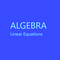Algebra simplified into a brief but thorough free resource can benefit both a parent helping a student and the student. This segment addresses linear equations.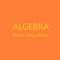This is the second part of a free resource to help with the basic understqanding of algebra. It can be used by students and those helping students witht the understanding.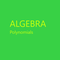This of a free resource where insights into algebra are provided to help both students and those helping students with algebra.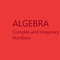This is a free to use algebra resource. It is designed to make it easy to understand complex and imaginary numbers for students and those assisting students with learning.

This article contains links to affiliate programs and Adsense advertising.  These must use cookies to allow for proper crediting, and allow me to earn from qualifying purchases. As an Amazon Associate I earn from qualifying purchases.

Updated: 02/09/2021, blackspanielgallery
2

Only logged-in users are allowed to comment. Login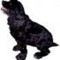blackspanielgallery on 05/03/2021

Simple reviews that do not bog down in complexities unique to one problem can refresh what has been learned.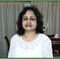WriterArtist on 05/03/2021

It is refreshing to go through Algebra I thought was forgotten. Fortunately I found that I remembered everything when I read your article.blackspanielgallery on 02/12/2021

I have taught algebra so many times I just make them up as I go. Experience allows me to know the variety of examples needed, and I try for a good coverage.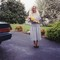DerdriuMarriner on 02/12/2021

blackspanielgallery, Thank you for the practicalities and the products.
How do you choose the examples that you did here and in your other algebra series articles?

#### You might also like

Learn Algebra and Trigonometry with Rap Videos

Learn the hard to grasp Algebra and Trigonometry basics from inspiring math t...

The Retired Professor Simplifies Algebra: Systems of Equations

Insights into algebra with attention to systems of linear equations is the fo...

The Retired Professor Simplifies Algebra: Factoring

This algebra insight is intended to assist students and those helping student...

Disclosure: This page generates income for authors based on affiliate relationships with our partners, including Amazon, Google and others.